Sample Data: Abalone Measurements

Predict the age of abalone from physical measurements

Traditionally, the age of abalone is determined by cutting the shell through the cone, staining it, and counting the number of rings through a microscope. These data were collected to determine whether the age of abalone could instead be determined using the following physical measurements: sex, length, diameter, height, whole weight, shucked weight, viscera weight, and shell weight.

Examples

Basic Examples

Retrieve the resource:

 In:=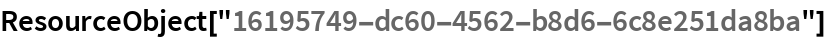Out=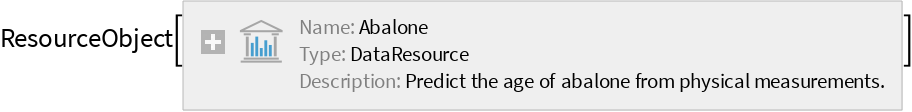Retrieve the default content:

 In:=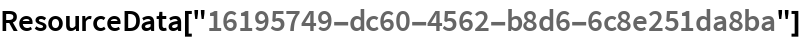Out=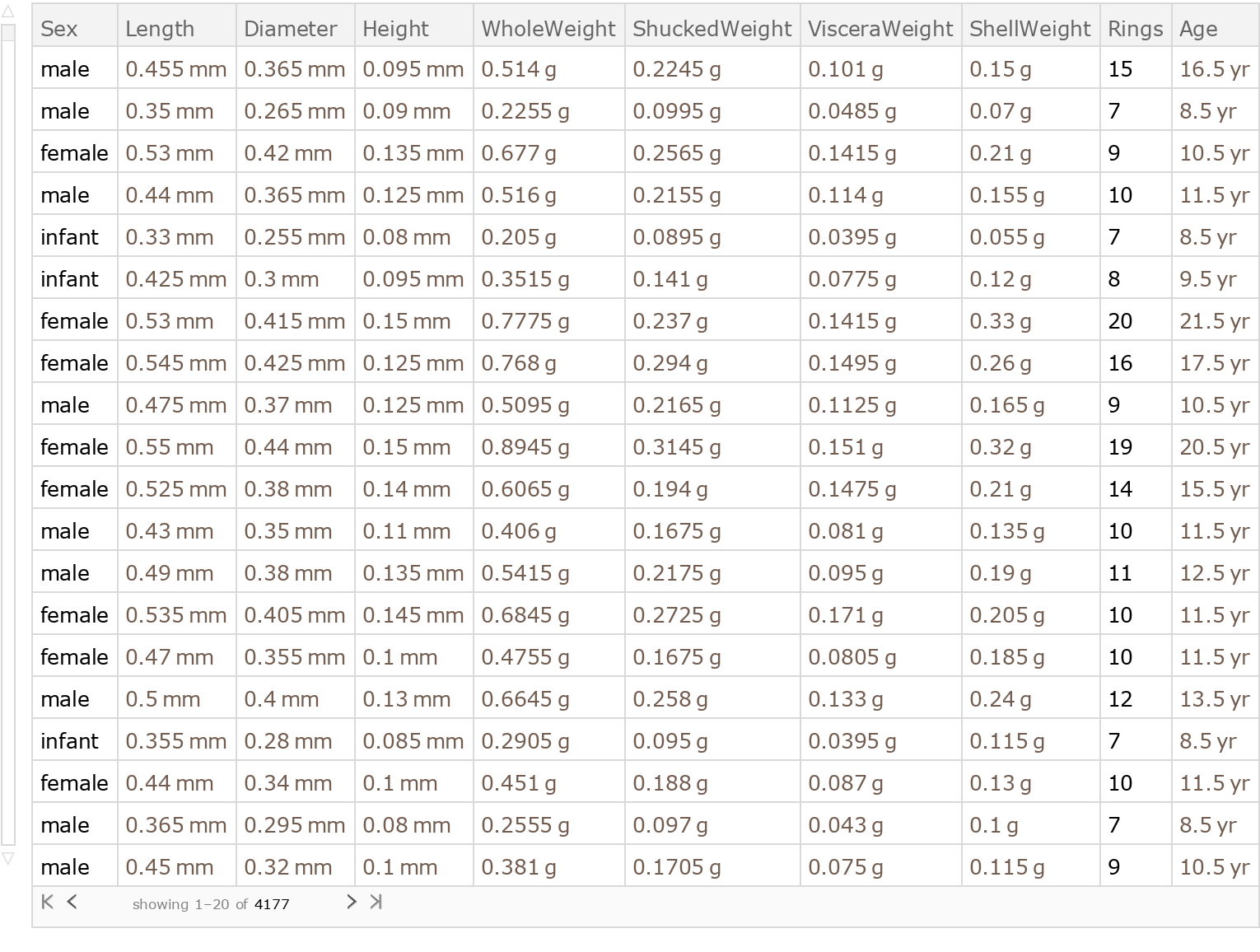Analysis

Shuffle the dataset randomly, remove the unit labels and drop the age column:

 In:=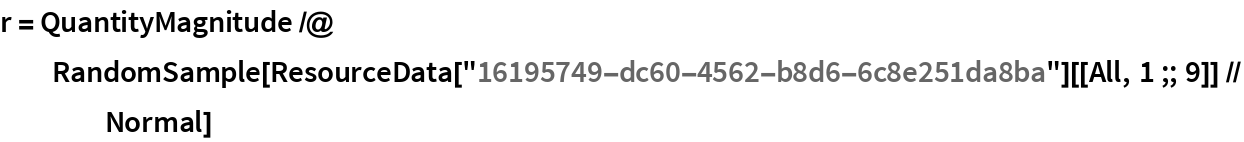Out=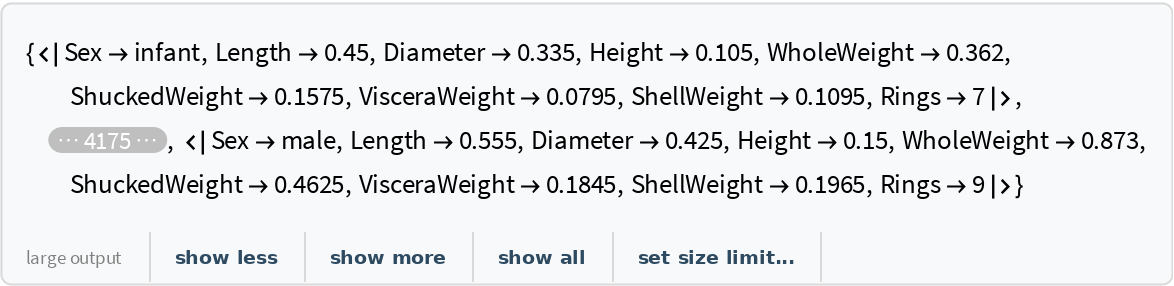Create a training dataset using 80% of the original dataset:

 In:=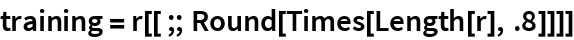Out=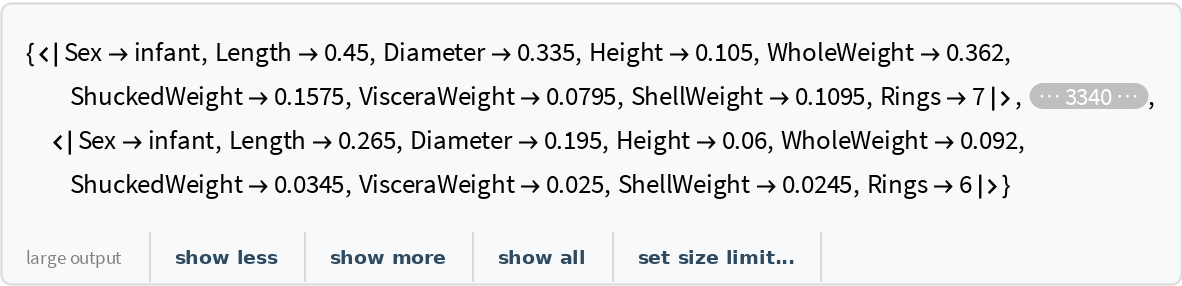Create a testing dataset using the remaining 20% of the original dataset:

 In:=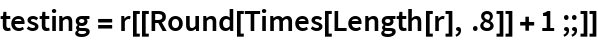Out=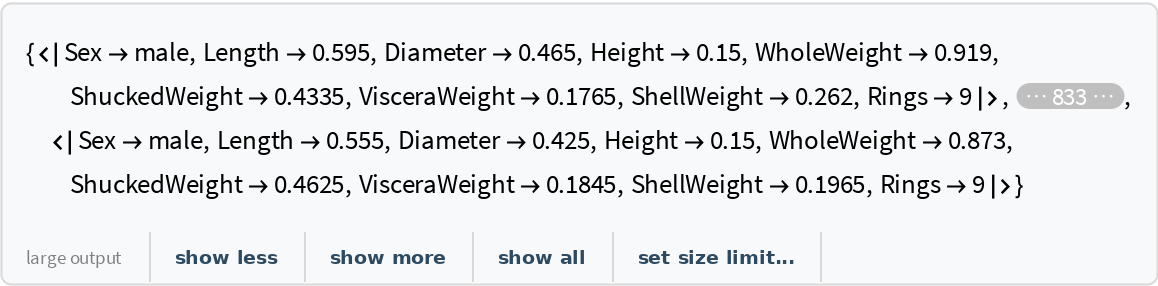Train a predictor function using "Rings" as the target variable:

 In:=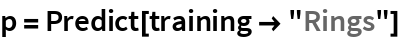Out=Obtain general information about the predictor:

 In:=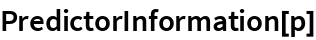Out=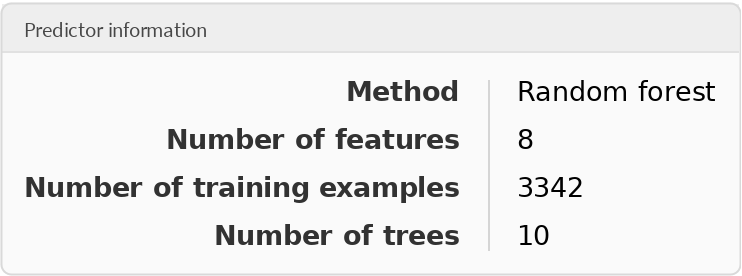Test the predictor:

 In:=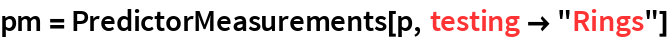Out=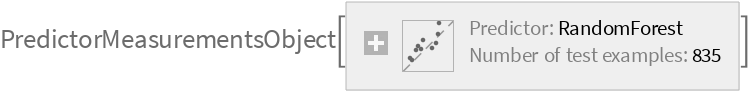Visualize the accuracy of the predictor:

 In:=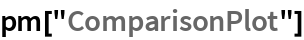Out=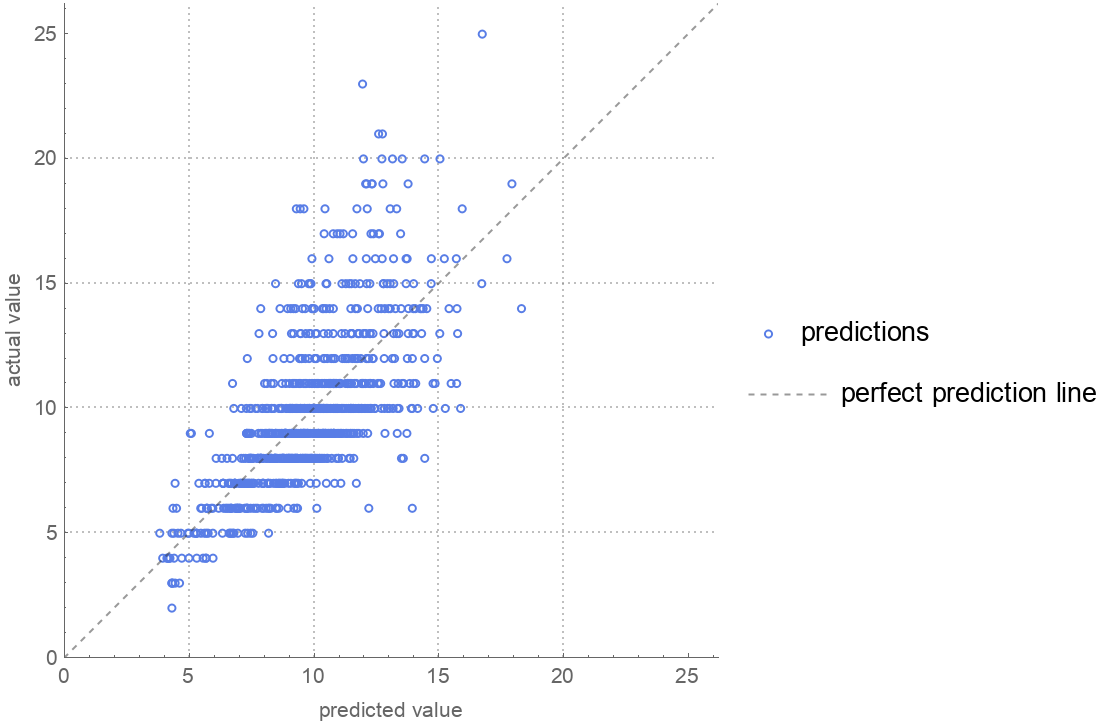Wolfram Research, "Sample Data: Abalone Measurements" from the Wolfram Data Repository (2017)